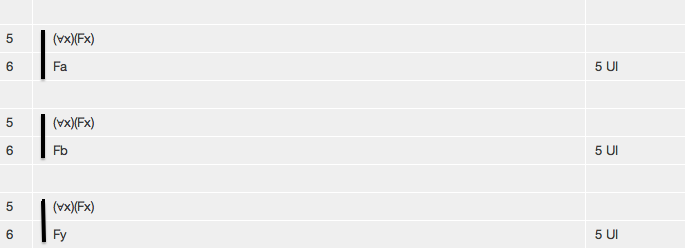# Tutorial 18: Simplified Universal Instantiation

Logical System

2013

### Skill to be acquired:

To understand the concepts of scope, free, bound. To meet substitution and a simplified version of the rule for removing a Universal Quantifier.

### The Tutorial

There is a new rule of derivation-- Universal Instantiation-- which, roughly speaking, allows you to do the following... if a formula has a Universal Quantifier as its main connective you may remove the Universal Quantifier and have as a new line the body of the formula with a term substituted in for all free occurrences of the variable of quantification within the scope. For example,are all proof fragments illustrating Universal Instantiation.

There is a restriction on the rule, but to understand the restriction you need to understand the concepts which are used to express it.

Notice the form of a formula with a quantifier as its main connective. Examples of such formulas are (∀x)(Fx), (∃x)(Fx∧Gx), (∀x)(Fx⊃Gx). In each case there is the quantifier with its variable, enclosed within brackets, and this is followed by a formula known as the scope of the quantifier. In our examples, (Fx) is the scope of the first one, (Fx∧Gx) the scope of the second, and (Fx⊃Gx) the scope of the third.

More formally...

If <scope> is any well-formed formula then (∀<variable>)<scope> is the universal quantification of <scope> using <variable> and (∃<variable>)<scope> is the existential quantification of <scope> using <variable> . In each case the <scope> is the scope of the quantification.

The notion of the scope of a quantifier is important; and it is good practice to enclose the scope in brackets so as to display exactly what it is. For example, the scope of the formula (∀x)Fx⊃Gx is Fx not (Fx⊃Gx); the formula as a whole will be read as ((∀x)(Fx))⊃Gx; such a formula is better written as (∀x)(Fx)⊃Gx.

A quantifier binds all occurrences of its <variable> in its scope. An occurrence of a variable which is not bound is called free. So, in (∀x)(Fx)⊃Gx the occurrence of x immediately after F is bound whereas the occurrence of x immediately after G is free; but in the formula (∀x)(Fx⊃Gx) both occurrences are bound.

Substitutions are on occasions made of one term for free occurrences of another-- in Universal Instantiation, for example.The notation <wff>[<newterm>/<oldterm>] means the formula <wff> with <newterm> substituted for the free occurrences of <oldterm> throughout. So, for example, (Fx⊃Gx)[a/x] is (Fa⊃Ga).

There is an obstacle to successful substitution, and that is capturing. The best examples of this disastrous phenomenon use relations, which is a topic we do not encounter until Tutorial 23. We will leave discussion of it until then.

The full rule of Universal Instantiation needs to take account of capturing, but we can give a simplified version of the rule here.

#### The Simplified Rule of Universal Instantiation UI

If a derivation contains a line of the form

n (∀<variable>)<scope> <any justification>

then a line of the form

<<scope>[<term>/<variable>]> 'n UI'

may be added to the derivation, provided that the <term> to be substituted in either is a constant term or a variable new to the <scope>.

## Exercise to accompany Predicate Tutorial 8

### Exercise 1 (of 3)

Identify the scopes of the following formulas

a) (∀x)(Fx)
b) (∀x)(Fx⊃Gx)
c) (∀x)(Fx)⊃Gx
d) (∃x)(Fx)∧Gx
e) (∃x)(Fx∧Gx)
f) (∀y)(Hy⊃(∃x)(Fx∧Gx))
g) (∀y)((∀x)((∀z)(Hy∧(Fz∧Gx))))

a) (Fx)

b) (Fx⊃Gx)

c) This is a bit of a trick question, sorry. (∀x)(Fx)⊃Gx has as its main connective the ⊃ so the formula as a whole is not a quantified formula and does not have a scope. However, if our interest is with the quantified formula (∀x)(Fx) which is a subformula of (∀x)(Fx)⊃Gx then it has scope (Fx).

d) This also is a trick. (∃x)(Fx)∧Gxhas as its main connective the ∧ so the formula as a whole is not a quantified formula and does not have a scope. However, if our interest is with the quantified formula (∃x)(Fx) which is a subformula of (∃x)(Fx)∧Gx then it has scope (Fx).

e) (Fx∧Gx)

f) (Hy⊃(∃x)(Fx∧Gx))

g) ((∀x)((∀z)(Hy∧(Fz∧Gx))))

### Exercise 2 (of 3)

Identify the free and bound occurrences of the variable x in following formulas

a) (∀x)(Fx)
b) (Fx⊃Gx)
c) Fx⊃ (∀x)(Gx)
d) (∀x)(Fx)⊃Gx
e) (∃x)(Fx)∧Gx
f) (∃x)(Fx∧Gx)

a) (∀x)(Fxbound)

b) (Fxfree⊃Gxfree)

c) Fxfree⊃ (∀x)(Gxbound)

d) (∀x)(Fxbound)⊃Gxfree

e) (∃x)(Fxbound)∧Gxfree

f) (∃x)(Fxbound∧Gxbound)

### Exercise 3 (of 3)

Derive the following valid arguments

(a) (∀x) (Fx) ∴ Fa
(b) (∀x) (Fx) ∴ Fa∧Fb
(c) Fc,(∀x) (Fx⊃Gx) ∴ Gc
(d) Fa,(∀x) (Fx⊃Gx), (∀x) (Gx⊃Hx) ∴Ha
(e) (∀x) (Fx⊃Gx), (∀x) (Gx⊃Hx) ∴(Fa⊃Ha)